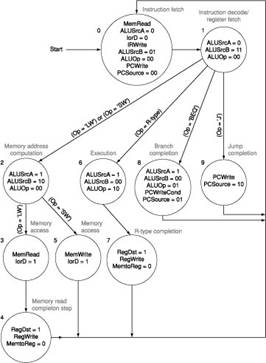Computer Organization and Structure

Homework #4

Due: 2008/12/9

1.      Describe the effect that a single stuck-at-n fault (i.e., regardless of what it should be, the signal is always n, where n = 0 or 1) would have for the signals in the following sub-questions:

a.         For single stuck-at-0 fault, which instructions, if any, will not work correctly in the single-cycle datapath as shown in Figure 1? Explain why. Consider each of the following faults separately:

RegWrite = 0

ALUop0 = 0

ALUop1 = 0

Branch = 0

MemWrite = 0Figure 1: The simple datapath with the control unit.

b.        For single stuck-at-1 fault, which instructions, if any, will not work correctly in the single-cycle datapath? Explain why.

c.         For single stuck-at-0 fault, which instructions, if any, will not work correctly in the multiple-cycle datapath as shown in Figure 2? Explain why. Consider each of the following faults separately:

a)      RegWrite = 0

c)      MemWrite = 0

d)     IRWrite = 0

e)      PCWrite = 0

f)       PCWriteCond = 0Figure 2: The multicycle datapath with the control lines.

d.        For single stuck-at-1 fault, which instructions, if any, will not work correctly in the multiple-cycle datapath? Explain why.

2.      MIPS chooses to simplify the structure of its instructions. The way we implement complex instructions through the use of MIPS instructions is to decompose such complex instructions into multiple simpler MIPS ones. Show how MIPS can implement the instruction swap \$rs, \$rt, which swaps the contents of registers \$rs and \$rt. Consider the case in which there is an available register that may be destroyed as well as the care in which no such register exists. If the implementation of this instruction in hardware will increase the clock period of a single-instruction implementation by 10%, what percentage of swap operations in the instruction mix would recommend implementing it in hardware?

3.      We wish to add the instructions jr (jump register), sll (shift left logical), lui (load upper immediate), and a variant of the lw (load word) instruction to the single-cycle datapath. The variant of the lw instruction increments the index register after loading word from memory. This instruction (l_inc) corresponds to the following two instructions:

lw       \$rs, L(\$rt)

Add any necessary datapaths and control signals to Figure 1 and show the necessary additions to Table 1. You can photocopy Figure 1 and Table 1 to make it faster to show the additions.

 Instruction RegDst ALUSrc Memto Reg Reg Write Mem Read Mem Write Branch ALUOp1 ALUOp0 R-format 1 0 0 1 0 0 0 1 0 lw 0 1 1 1 1 0 0 0 0 sw X 1 X 0 0 1 0 0 0 beq X 0 X 0 0 0 1 0 1

Table 1: The setting of the control lines is completely determined by the opcode fields of the instruction.

4.      Show how the jump register (jr) instruction can be implemented simply by making changes to the finite state machine shown in Figure 3. (It may help you to remember that \$0=\$zero=0.)Figure 3: The complete finite state machine control for the datapath shown in Figure 2.